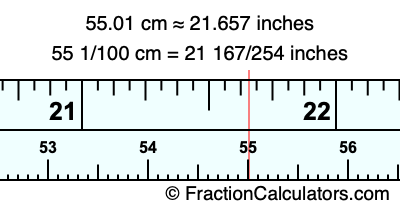55.01 cm in inchesHere is how to convert 55.01 cm to inches. We will give you the fractional answer, the decimal answer, and illustrate the answer on our tape measure.

One centimeter is equal to 50/127 inches, and 55.01 as a fraction is 55 1/100. Therefore, to get the fractional answer to 55.01 cm in inches, we multiply 55 1/100 by 50/127 and simplify if necessary.

cm × 50/127 = inches
55 1/100 × 50/127 = inches
55 1/100 × 50/127 = 21 167/254
55 1/100 cm = 21 167/254 inches
55.01 cm = 21 167/254 inches

As promised above, we also have the answer to 55.01 cm in inches in decimal form. Although the fractional answer above is exact, the decimal answer may be rounded if necessary:

21 167/254 ≈ 21.6574803149606
21 167/254 ≈ 21.657
55.01 cm ≈ 21.657 inches

Our image below shows 55.01 cm on a tape measure. The top row of the tape measure is inches, and the bottom row is centimeters.As you can see, we drew a red line where 55.01 cm and 21.657 inches meet on the measuring tape.

Centimeters to Inches Calculator
Here you can convert another length in centimeters to inches.

Convert  cm to inches.

What is 55.02 cm in inches?
Here is another inches to centimeters calculation we did for you.